Tamil Nadu Board of Secondary EducationSSLC (English Medium) Class 10th

# Concept of Prism

#### definition

• Prism: A prism is a polyhedron whose base and top are congruent polygons and whose other faces, i.e., the lateral faces are parallelograms, rectangles, or squares. All prism is polyhedron, which means all faces are flat.

# Prism:

A prism is a polyhedron whose base and top are congruent polygons and whose other faces, i.e., the lateral faces are parallelograms, rectangles, or squares. All prism is polyhedron, which means all faces are flat.

## Properties of a prism:

• The two parallel and congruent faces of a prism are called bases. The other faces of a prism are called lateral faces.

• The number of lateral faces in a prism is the same as the number of sides of its polygonal bases.

• The line segments created by two intersecting faces are called edges.

• The vertices are points where three or more edges meet.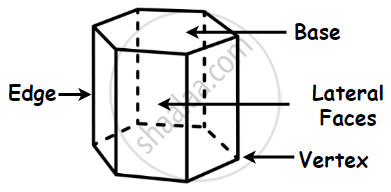• If the base of a prism is a regular polygon, it is called a regular prism. Otherwise, it is an irregular prism.

### Irregular Prism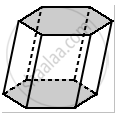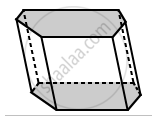The bases for the regular hexagonal prism are regular hexagons.

The bases for the hexagonal prism are irregular hexagon.

## 2. Types of Prism:

### 1. Triangle Prism: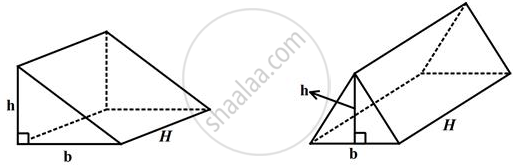• A right triangular prism has rectangular sides A triangular prism is composed of two triangular bases and three rectangular sides. It is a polyhedron made of a triangular base.

• A right triangular prism has rectangular sides, otherwise, it is oblique.

• A uniform triangular prism is a right triangular prism with equilateral bases and square sides.

• A triangular prism looks like the shape of a Kaleidoscope. It has triangles as its bases.

### 2. Square Prism: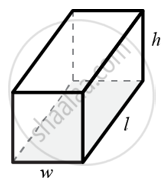A square prism is a cuboid (a convex polyhedron) which has 6 faces out of which two end-faces are square, 4 lateral faces are rectangles.

### 3. Pentagonal Prism: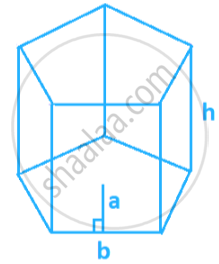The pentagonal prism is a prism having two pentagonal bases and five rectangular sides. It is a heptahedron with 7 faces, 15 edges, and 10 vertices.

### 4. Hexagonal Prism: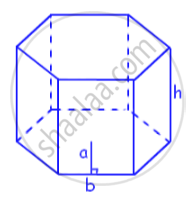The hexagonal prism has a total of 8 faces. It has 6 lateral faces that are parallelograms and 2 bases that are hexagons. It also has 18 edges and 12 vertices.

### 5. Rectangular Prism: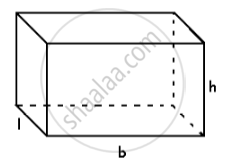• A rectangular prism is a solid, 3-dimensional object with six faces that are rectangles.

• It has the same cross-section along a length, which makes it a prism. The cross-section is a rectangle.

• A rectangular prism has 8 vertices, 12 sides, and 6 rectangular faces.

### 6. Right prisms and oblique prisms:

Prisms can also be further classified based on how their lateral faces intersect with their bases. If all the lateral faces are perpendicular to the bases, the prism is called a right prism. If not, it is called an oblique prism.

###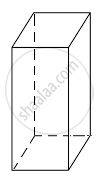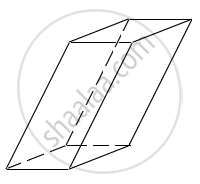If you would like to contribute notes or other learning material, please submit them using the button below.

Prism [00:12:30]
S
0%